$$\def\RR{\bf R} \def\real{\mathbb{R}} \def\bold#1{\bf #1} \def\d{\mbox{Cord}} \def\hd{\widehat \mbox{Cord}} \DeclareMathOperator{\cov}{cov} \DeclareMathOperator{\var}{var} \DeclareMathOperator{\cor}{cor} \newcommand{\ac}{\left\{#1\right\}}$$

## Pathway Lasso: Estimate Brain Information Flow Pathways

### Xi (Rossi) Luo

Brown University
Department of Biostatistics
Center for Statistical Sciences
Computation in Brain and Mind
Brown Institute for Brain ScienceNESS at Yale University
April 22, 2016

Funding: NSF/DMS (BD2K) 1557467; NIH P20GM103645, P01AA019072, P30AI042853; AHA

## CoauthorYi Zhao
(3rd Yr PhD Student)
Brown University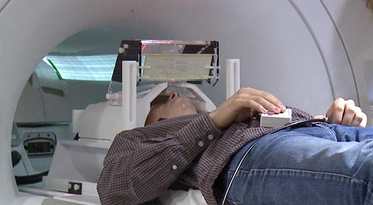• Task fMRI: performs tasks under brain scanning
• Story vs Math task: listen to story (treatment stimulus) or math questions (control), eye closed
• Not resting-state: "rest" in scanner

Goal: how brain processes story/math differently?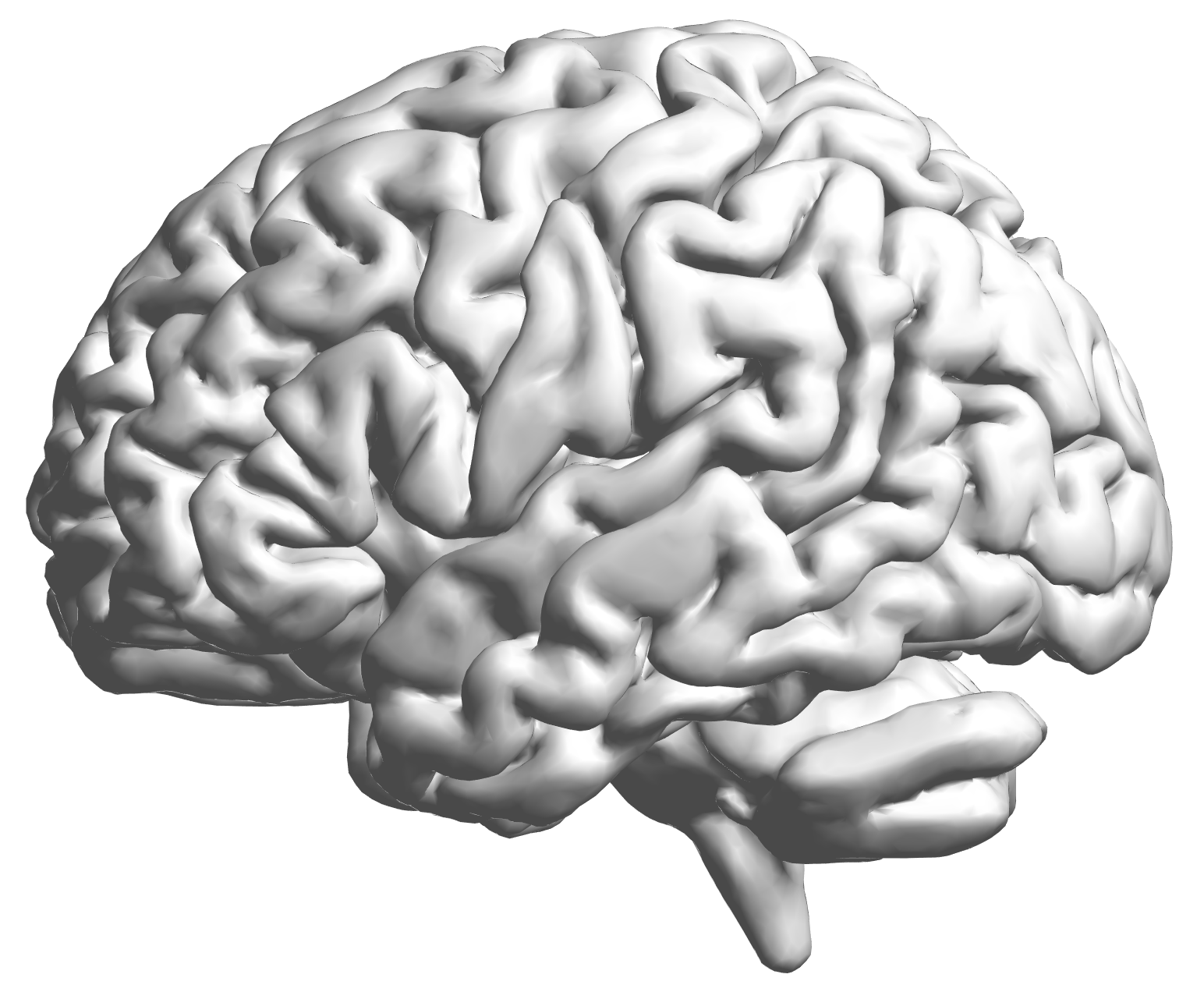fMRI data: blood-oxygen-level dependent (BOLD) signals from each cube/voxel (~millimeters), $10^5$ ~ $10^6$ voxels in total.

## fMRI Studies

Sub 1, Sess 1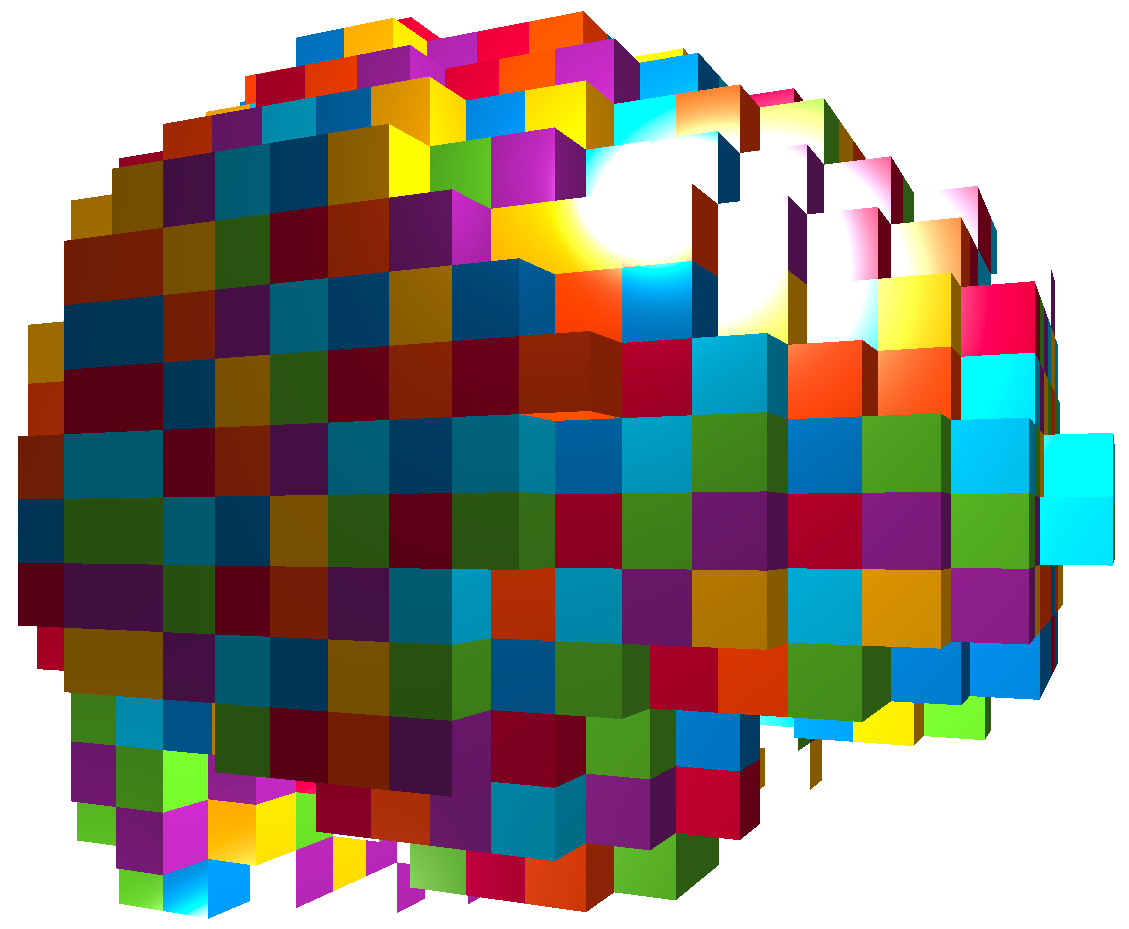Time 12~200

Sub i, Sess jSub ~100, Sess ~4This talk: one subject, two sessions (to test replicability)

## Network Model with Stimulus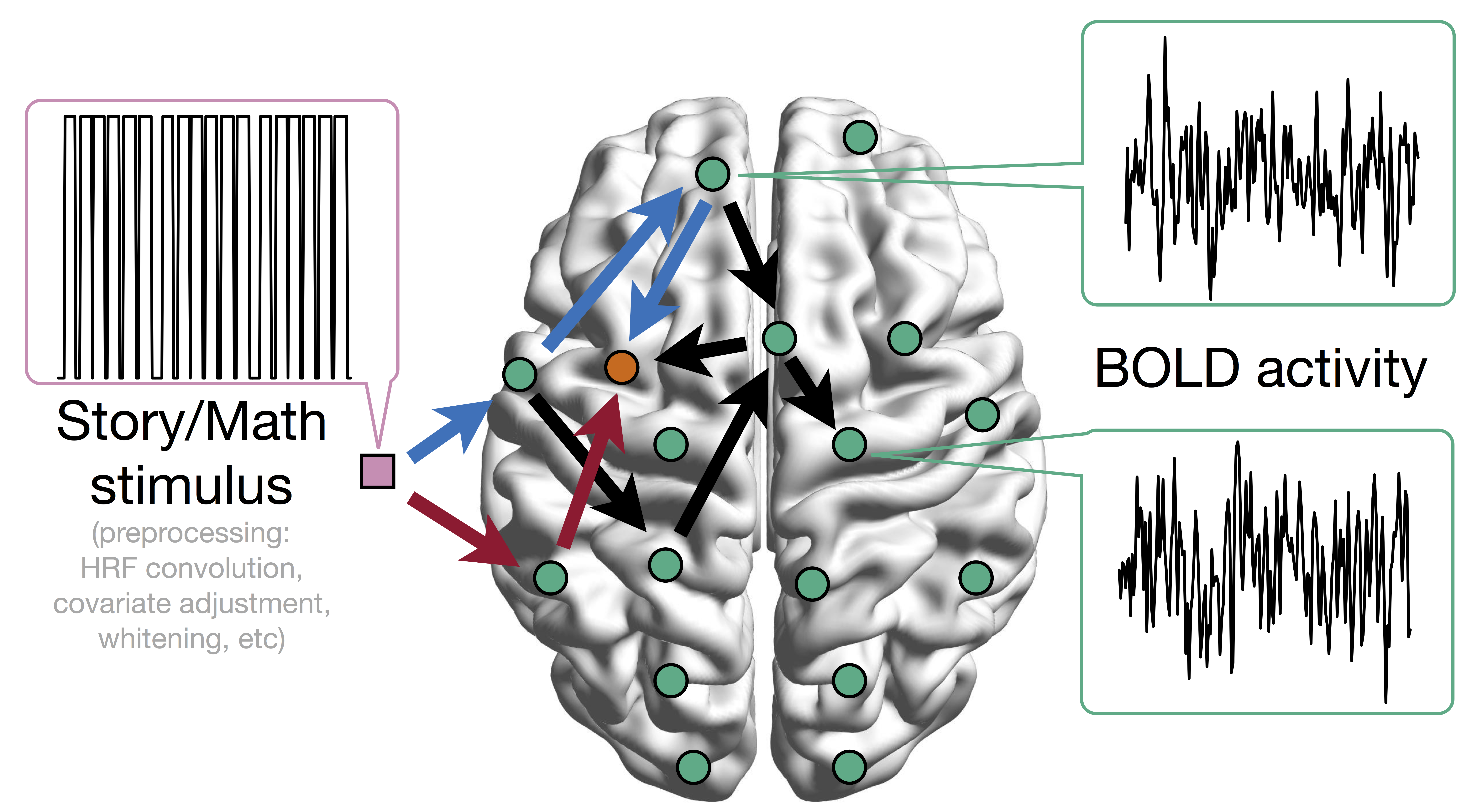Question: quantify red, blue, and other pathways
from stimulus to orange outcome circle/region Heim et al, 09

## Pathway=Activation+Connectivity

• Activation: stimulus $\rightarrow$ brain region activity
• Connectivity: one brain region $\rightarrow$ another region
• Whether not two or more brain regions "correlate"
• Pathway: stimulus $\rightarrow$ brain region A $\rightarrow$ region B
• Strong path: strong activation and strong conn
• Zero path: zero activation or zero conn, including
• Zero activation + strong conn = zero
• Strong activation + zero conn = zero

# Model

## Mediation Analysis and SEM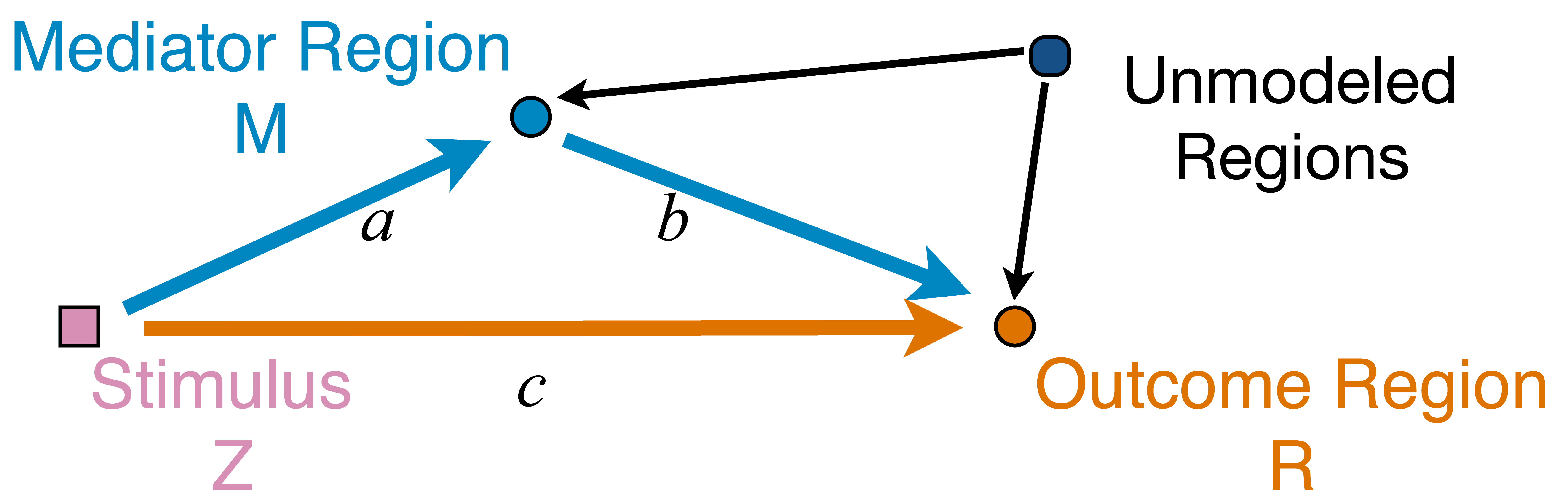\begin{align*}M &= Z a + {U + \epsilon_1}\\ R &= Z c + M b + {U g + \epsilon_2}\end{align*}
• Pathway effect: $a \times b$indirect; residual: $c$direct
• Mediation analysis
• Baron&Kenny, 86; Sobel, 82; Holland 88; Preacher&Hayes 08; Imai et al, 10; VanderWeele, 15;...

## Mediation Analysis in fMRI

• Parametric Wager et al, 09 and functional Lindquist, 12 mediation, under (approx.) independent errors
• Stimulus $\rightarrow$ brain $\rightarrow$ user reported ratings, one mediator
• Usual assumption: $U=0$ and $\epsilon_1 \bot \epsilon_2$
• Parametric and multilevel mediation Yi and Luo, 15, with correlated errors for two brain regions
• Stimulus $\rightarrow$ brain region A $\rightarrow$ brain region B, one mediator
• Correlations between $\epsilon_1$ and $\epsilon_2$
• This talk: multiple mediator and multiple pathways
• High dimensional: more mediators than sample size
• Alt: dimension reduction by arXiv1511.09354 Chen, Crainiceanu, Ogburn, Caffo, Wager, Lindquist, 15

## Full Pathway Model• Stimulus $Z$, $K$ mediating brain regions $M_1, \dotsc, M_K$, Outcome region $R$
• Strength of activation ($a_k$) and connectivity ($b_k$, $d_{ij}$)
• Too complex, even for small $K = 2$ Daniel et al, 14

## Reduced Pathway Model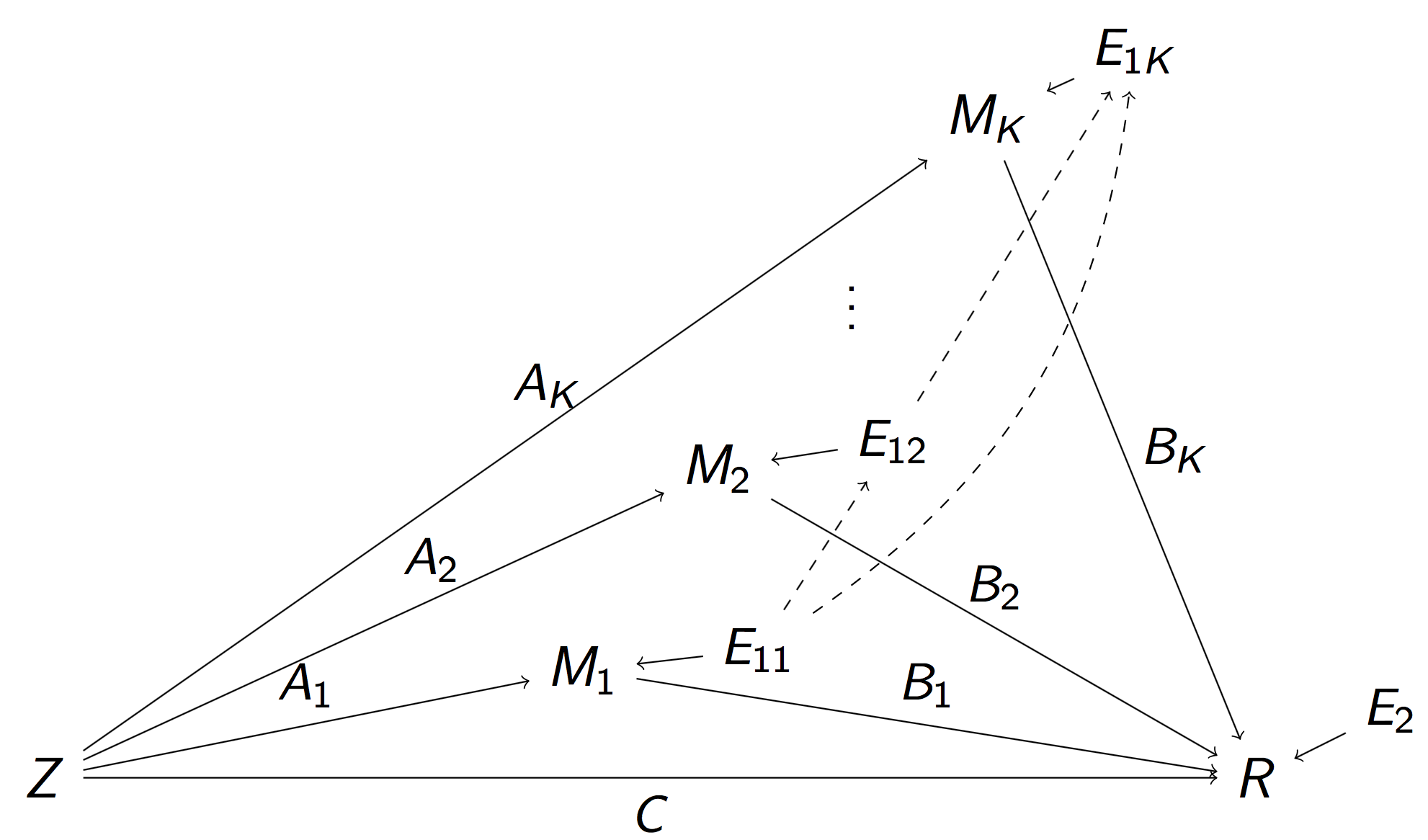\begin{align}M_k & = Z A_k + E_{1k},\, k=1,\dotsc, K\\ R & = Z C + \sum_{k=1}^{K} M_k B_k + E_2 \end{align}
• $A_k$: total inflow to mediator $M_k$; $B_k$: total conn
• Pathway effect: $A_k \times B_k$; Residual: $C$

## Relation to Full Model

• Proposition: Our "total" parameters has explicit forms of "individual" flow parameters in the full model
• Proposition: Our $E_k$'s are correlated, but won't affect estimation (will affect variance)
• Reduced model: a step to select spatial mediators
• Strong overall inflow and strong conn flow
• Favor reduced: challenging to determine the temporal order because of low temporal resolution

# Method

## Regularized Regression

• Minimize the penalized least squares criterion
$$\sum_{k=1}^K \| M_k - Z A_k \|_2^2 + \| R - Z C - \sum_k M_k B_k \|_2^2 + \mbox{Pen}(A, B)$$
The choice of penalty $\mbox{Pen}(\cdot)$ to be discussed
• All data are normalized (mean=0, sd=1)
• Want to select sparse pathways for high-dim $K$
• Alternative approach: two-stage LASSO Tibshirani, 96 to select sparse inflow and connection separately: $$\sum_{k=1}^K \| M_k - Z A_k \|_2^2 + \lambda \sum_k | A_k | \\ \| R - Z C - \sum_k M_k B_k \|_2^2 + \lambda \sum_k |B_k|$$

## Penalty: Pathway LASSO

• Select strong pathways effects: $A_k \times B_k$
• TS-LASSO: shrink to zero when $A$&$B$ moderate but $A\times B$ large
• Penalty (prototype) $$\lambda \sum_{k=1}^K |A_k B_k|$$
• Non-convex in $A_k$ and $B_k$
• Computationally heavy and non-unique solutions
• Hard to prove theory
• We propose the following general class of penalties$$\lambda \sum_{k=1}^K ( |A_k B_k| + \phi A_k^2 + \phi B_k^2)$$
Theorem $$v(a,b) = |a b| + \phi (a^2 + b^2)$$ is convex if and only if $\phi\ge 1/2$. Strictly convex if $\phi > 1/2$.

Contour Plot of Different Penalties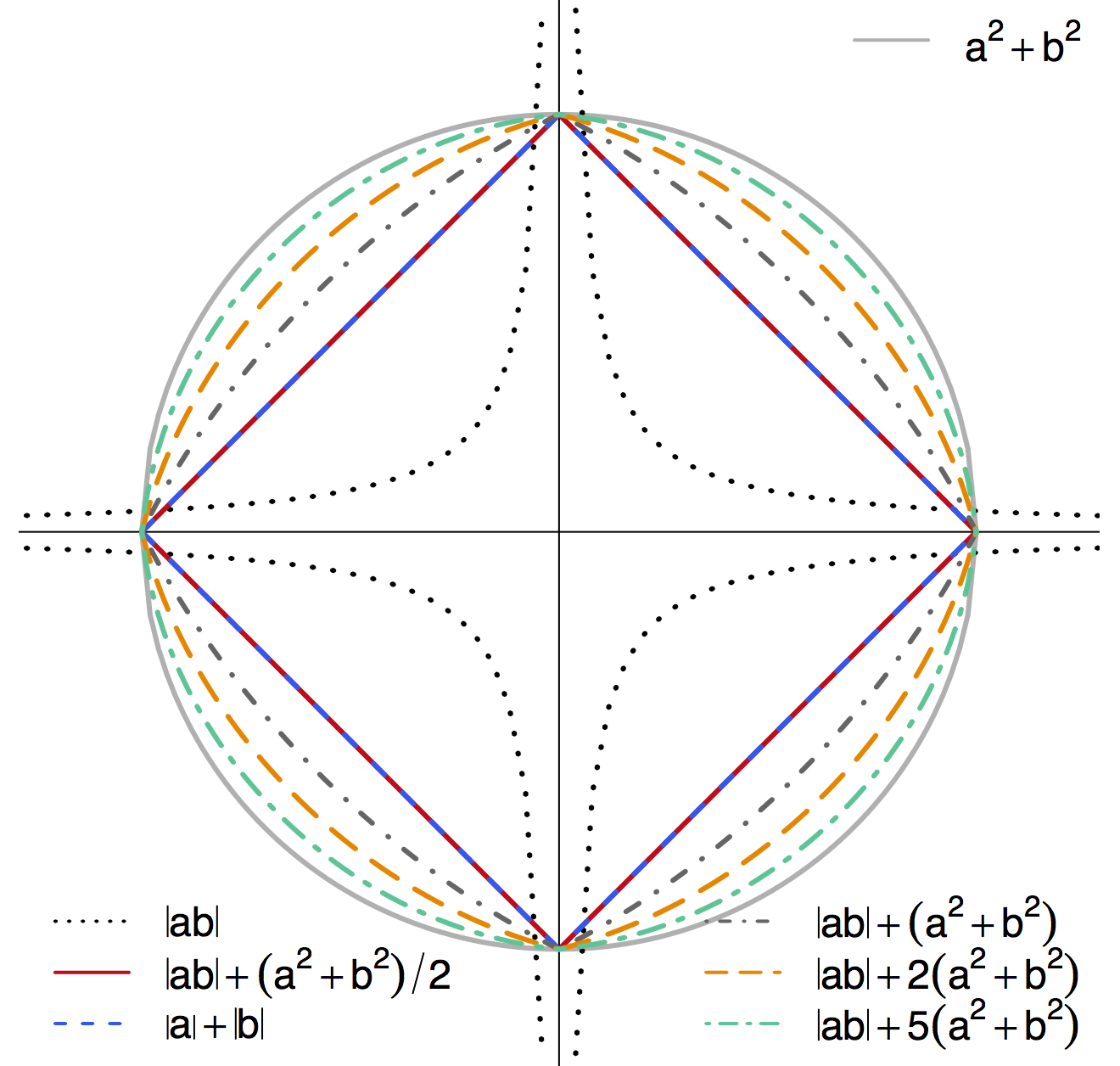• Non-differentiable at points when $a\times b = 0$
• Shrink $a\times b$ to zero
• Special cases: $\ell_1$ or $\ell_2$
• TS-LASSO: different $|ab|$ effects though $|a|+|b|$ same

## Algorithm: ADMM + AL

• SEM/regression loss: $u$; Non-differnetiable penalty: $v$
• ADMM to address differentiability \begin{aligned} \text{minimize} \quad & u(\Theta,D)+v(\alpha,\beta) \\ \text{subject to} \quad & \Theta=\alpha, \\ & D=\beta, \\ & \Theta e_{1}=1, \end{aligned}
• Augmented Lagrangian for multiple constraints
• Iteratively update the parameters
• We derive theorem on explicit (not simple) updates

## Complications

• Mixed norm penalty $$\mbox{PathLasso} + \omega \sum_k (|A_k| + |B_k|)$$
• Tuning parameter selection by cross validation
• Reduce false positives via thresholding Johnston and Lu, 09
• Inference/CI: bootstrap after refitting
• Remove false positives with CIs covering zero Bunea et al, 10

# Simulations

• Our PathLasso compares with TSLasso
• Simulate with varying error correlations
• Tuning-free comparison: performance vs tuning parameter (estimated effect size)
• PathLasso outperforms under CV

## Pathway RecoveryROCF1 Score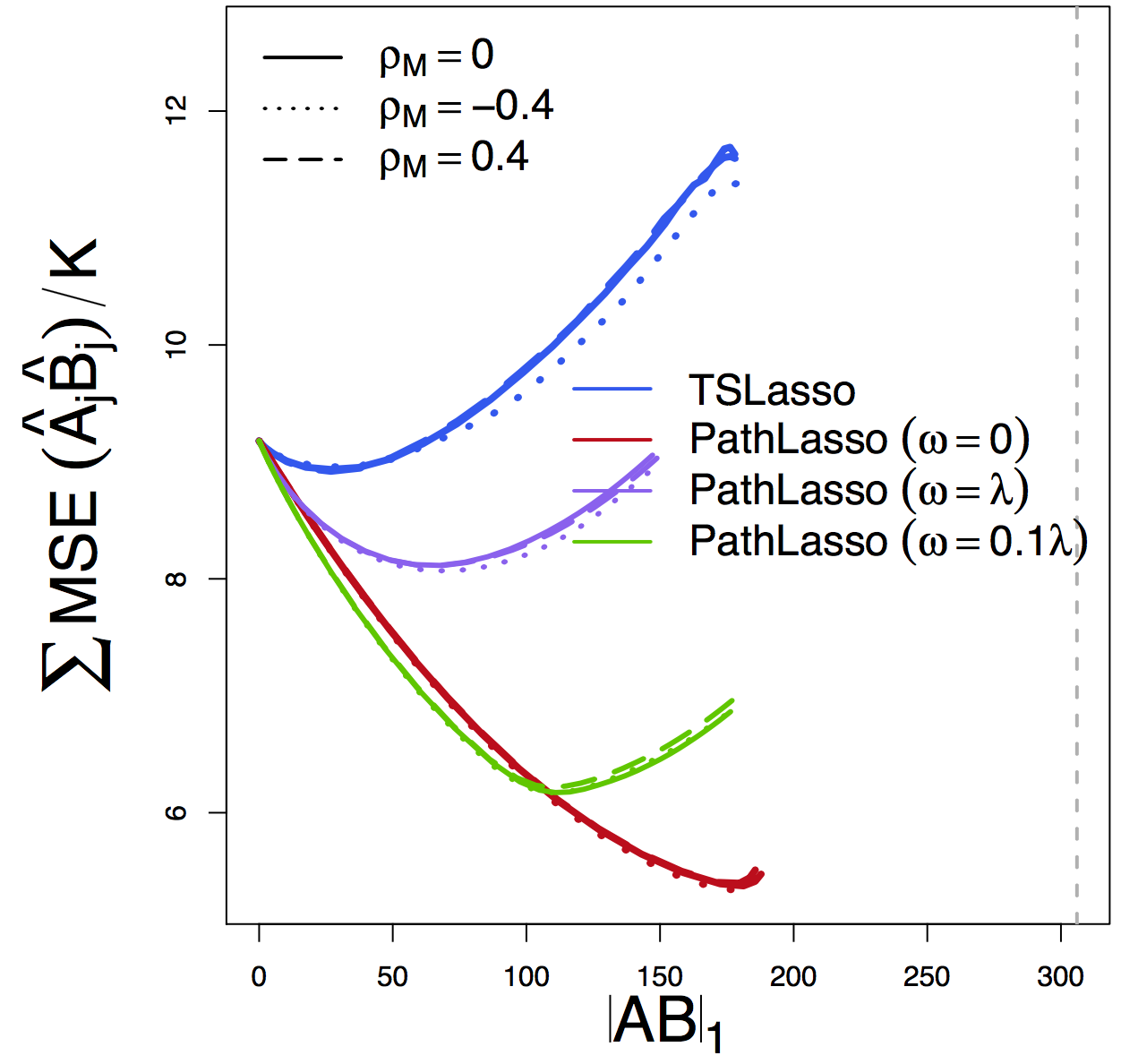MSE

Our PathLasso (red) outperforms two-stage Lasso (blue)

Other curves: variants of PathLasso and correlation settings

# Real Data: HCP

## Data: Human Connectome Project

• Two sessions (LR/RL), story/math task Binder et al, 11
• gICA reduces voxel dimensions to 76 brain maps
• ROIs/clusters after thresholding
• Apply to two sess separately, compare replicability
• Jaccard: whether selected pathways in two runs overlap
• $\ell_2$ diff: difference between estimated path effects
• Tuning-free comparisons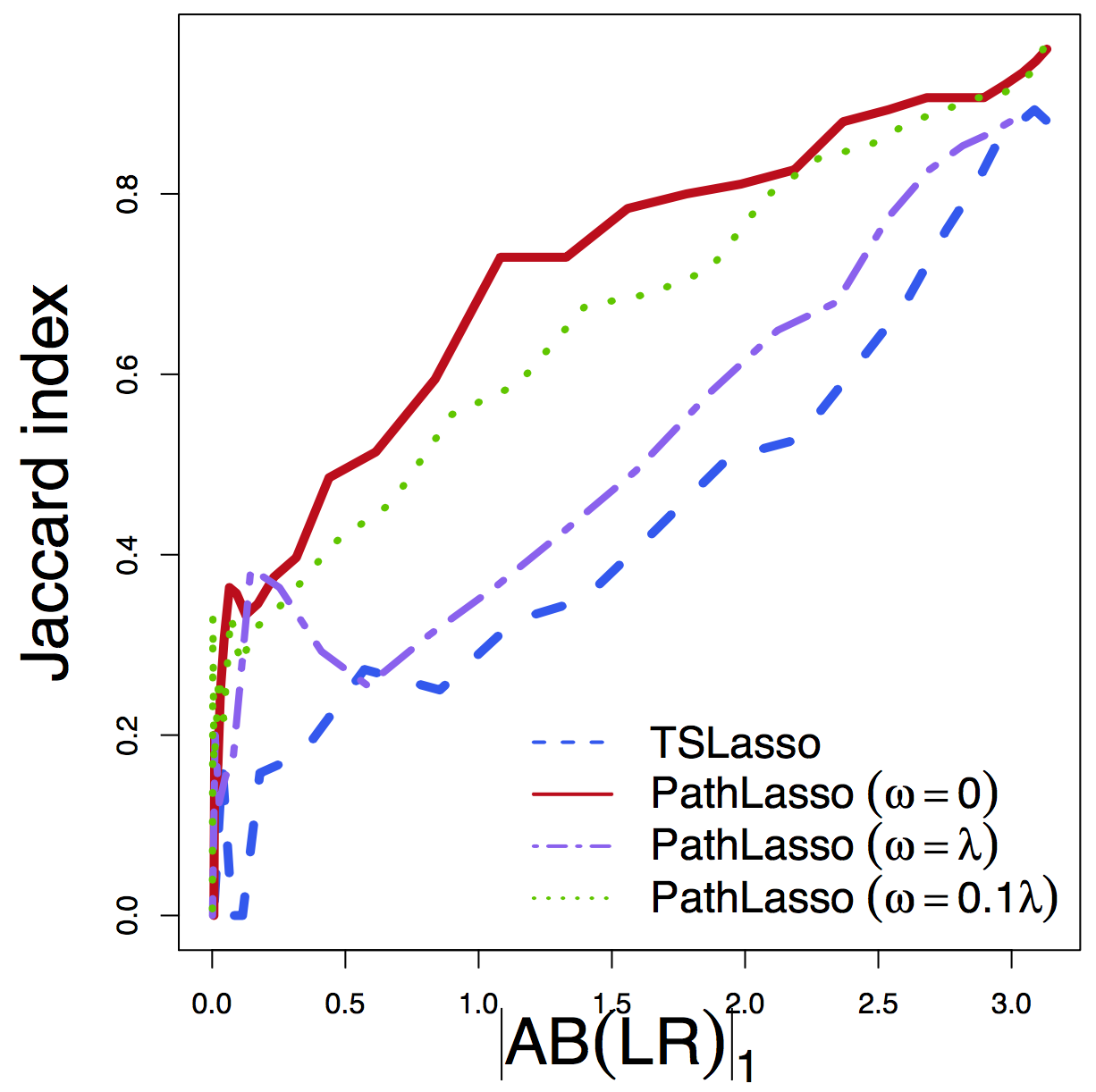Jaccard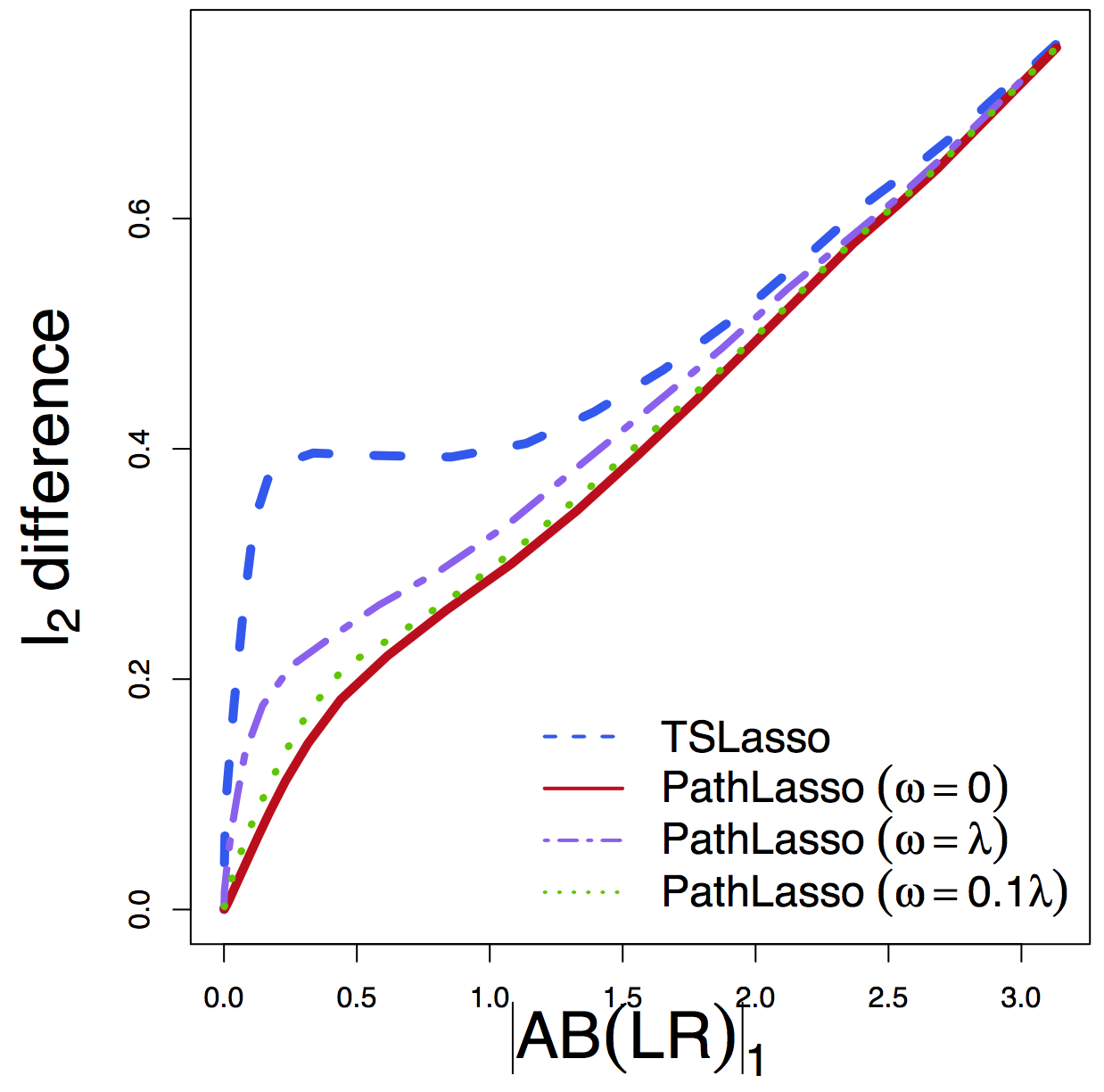$\ell_2$ Diff

Regardless of tuning, our PathLasso (red) has smaller cross-sess diff (selection and estimation) than TSLasso (blue)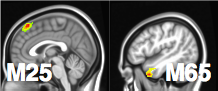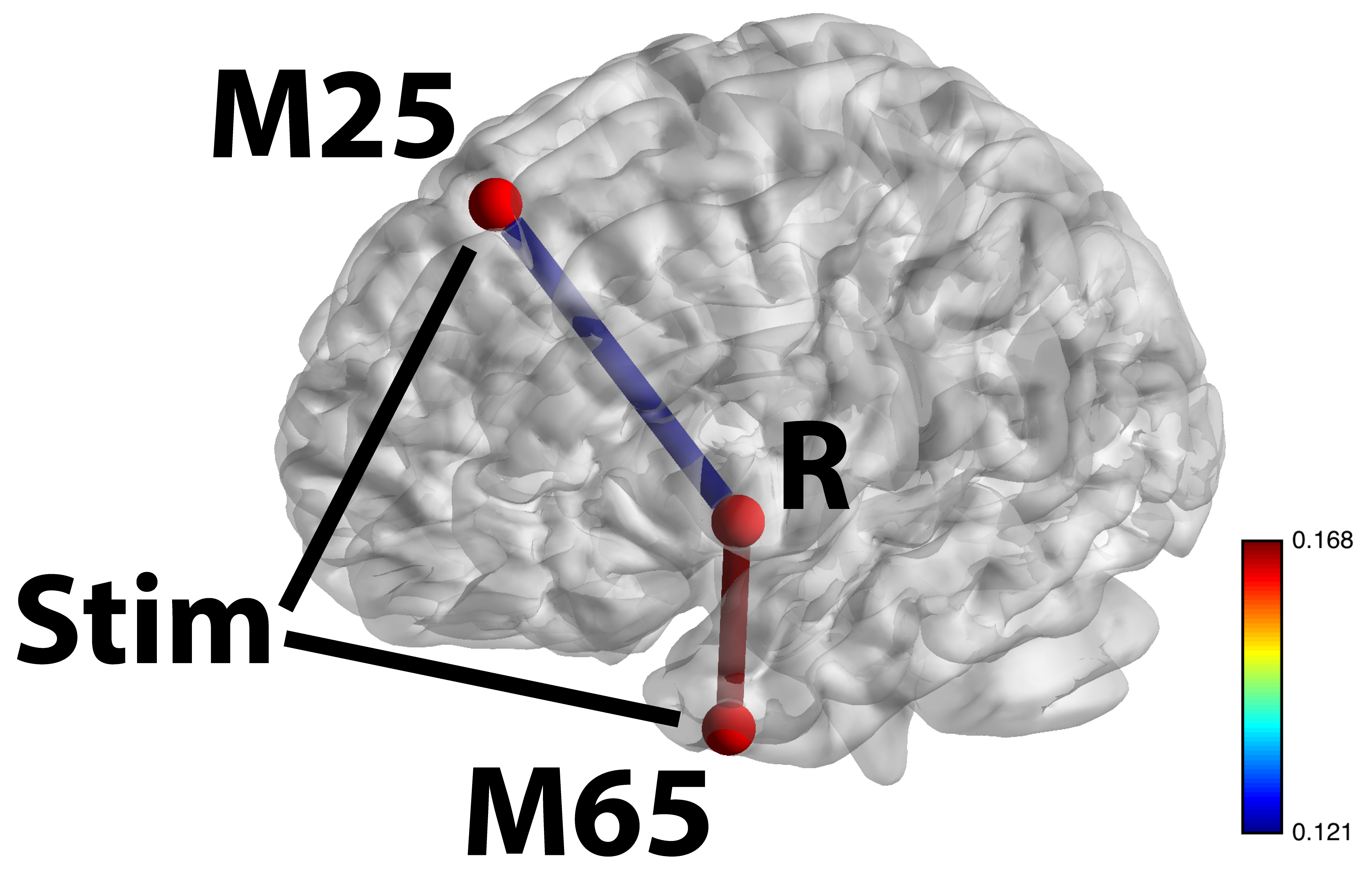Stim-M25-R and Stim-M65-R significant shown largest weight areas

• M65 responsible for language processing, larger flow under story
• M25 responsible for uncertainty, larger flow under math

## Summary

• High dimensional pathway model
• Penalized SEM for pathway selection and estimation
• Convex optimization for non-convex products
• Sufficient and necessary condition
• Algorithmic development for complex optimization
• Improved estimation and selection accuracy
• Higher replicability in HCP data
• Manuscript: Pathway Lasso (arXiv 1603.07749)

# Thank you!

## Slides at: bit.ly/xlNESS16